• 粒子群算法(PSO)初识

千次阅读 2018-12-12 13:10:52
粒子群算法PSO是模拟群体智能所建立起来的一种优化算法，用于解决各种优化问题。   抽象问题实例化： 假设一群 鸟在觅食，只有一个地方有 食物，所有鸟儿都看不见食物（不知道食物的具体位置，知道了就不无需...

粒子群算法PSO是模拟群体智能所建立起来的一种优化算法，用于解决各种优化问题。

抽象问题实例化：

假设一群 鸟在觅食，只有一个地方有 食物，所有鸟儿都看不见食物（不知道食物的具体位置，知道了就不无需觅食了）， 但是能闻见食物的气味，知道食物的 远近（即知道食物和自己的距离）。

假设鸟与鸟之间能共享信息（即互相知道每个鸟离食物多远，这个是人工设定鸟群合作），那么最好的策略就是结合自己离食物最近的位置和鸟群中其他鸟距离食物最近的位置这2个因素综合考虑找到最好的 搜索位置。

一、基本概念

PSO核心概念：

1.粒子（particle）:一只鸟。

2. 种群（population）：鸟群。

3. 位置（position）：一个粒子（鸟）当前所在的位置。

4. 经验（best）：一个粒子（鸟）自身曾经离食物最近的位置。

5. 速度（velocity）：一个粒子（鸟）飞行的速度。

6. 适应度（fitness）：一个粒子（鸟）距离食物的远近。

二、粒子算法的过程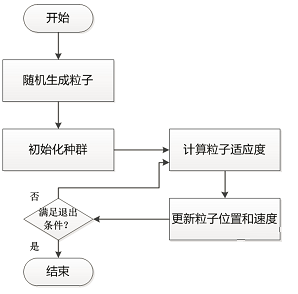粒子群算法步骤：

1）根据问题需要， 随机生成粒子，粒子的数量可自行控制。

2）将粒子组成一个种群。

3）计算粒子的适应度值。（即 ）计算粒子距离最优值得远近。

4）更新种群中每个粒子的位置和速度。

5）满足退出条件就退出，不满足 就转向步骤3）。

三、PSO核心——速度更新

从PSO算法流程图中可以看出，核心步骤是更新种群中每个粒子的位置和速度，而速度的更新是核心中的核心。

粒子速度更新公式如下：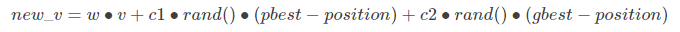v为粒子当前的速度，w为惯性因子（有速度就有运动惯性）。rand()为随机数生成函数，生成0~1之间的随机数。

position为粒子当前的位置，pbest为本粒子历史上最好的位置，gbest为种群中所有粒子中当前最好的位置。

c1和c2表示学习因子，分别向本粒子历史最好位置 和种群中当前最好位置学习。

注： 实际 中设置c1=c2=1，w=0.5

下面从物理原理上解释这个速度更新公式，速度更新公式分为3个部分：

第一部分是惯性保持部分，粒子沿着当前的速度和方向惯性飞行，不会偏移。（牛顿运动学第一定理）

第二部分是自我认识部分，粒子受到自身历史最好位置的吸引力，有回到自身最好位置的意愿。（牛顿运动学第二定理）

第三部分是社会认知部分，粒子处在一个社会中（种群中），社会上有更好的粒子（成功人士），粒子 受到成功人士的 吸引力，有去社会 中成功人士位置的意愿。（牛顿 运动学第二定理）

速度更新公式的物理含义：粒子在自身惯性和2中外力作用下，速度和方向发生改变。

这3部分都有重要含义。没有惯性部分，粒子们将很快向当前的自身最优位置和局部最优位置

靠拢，变成了一个局部算法。有了惯性，不同粒子有在空间中自由飞行的趋势，能够在整个

搜索区域内寻找最优解。而没有自我认知部分，粒子们将向当前的全局最优粒子位置靠拢，

容易陷入局部最优。没有社会认知部分，粒子们各自向自身最优位置靠拢，各自陷入自身最优

位置，整个搜索过程都不收敛了。

有了速度更新公式，位置更新就简单了：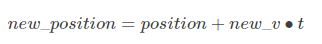注：t一般默认取1。

展开全文• 关于自适应粒子群算法的MATLAB代码，过程很详细，适合初学者学习。
• 第三章针对PSO算法求解组合优化问题时，速度迭代公式难以定义的问题，提出等值变换、异值变换和变换序列等概念的基础上，通过重新定义粒子的速度和位置迭代公式，设计随机自适应粒子群优化模型并用以求解0-1背包问题...粒子群算法
• 进化算法之粒子群（PSO）

千次阅读 2016-11-12 20:32:30
进化 优化 粒子群

概述：

正如其他的那些进化算法一样，粒子群算法的灵感同样来自于大自然。它由Eberhart和Kennedy共同提出的，其基本思想来自于他们早期对许多鸟类的群体行为进行建模仿真研究结果的启发。

假设在一块广袤无垠的栖息地上有一群自由自在的鸟儿和一堆丰盛的食物，可是鸟儿却不知道食物在哪，现在我们的目标是让所有的鸟儿找到这堆美食，那该怎么办呢？Kennedy认为鸟之间存在着相互交换信息，于是他们在仿真中添加了一些内容：每个个体（鸟儿）能够通过一定规则估计自身位子的适应值，并能记住自己当前所找到的最好位置，记作：“局部最优pBest”。此外还应记住整个群里中所有鸟儿找到的最优位置，记作：“全局最优gBest”。这两个最优变量使得鸟在某种程度上朝这些方向靠近。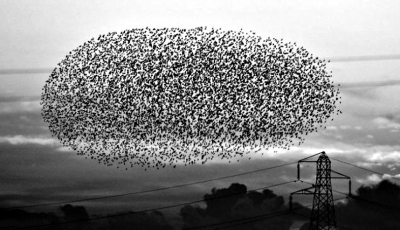基本数学概念：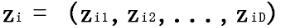为第i个粒子（i=1,2,3…m）的D维位置矢量，根据事先设定的适应值函数可以计算出Zi的当前适应值，即衡量粒子位置优劣的函数。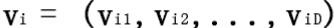为粒子i的飞行速度，即粒子的飞行距离。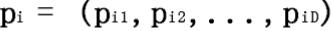为第i个粒子迄今为止搜索到的最优位置pBest。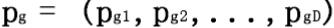为整个群的最优位置gBest。

在每次迭代中，粒子根据以下公式进行更新速度和位置：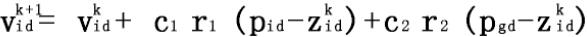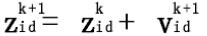其中i = 1,2,3…m；d = 1,2,3…D；k是迭代次数；r1和r2为[ 0 ，1]之间的随机数，为了保持群体的多样性；c1和c2为学习因子，也称加速因子，其使粒子具有自我总结和向群体中优秀个体学习的能力，从而向自己的历史最优点以及群体内最优点靠近，这两个参数对粒子群算法的收敛起的作用不是很大，但适当调整这两个参数可以减少局部最优的困扰。

粒子群算法的速度公式右边包含了3个部分：第一部分是粒子之前的速度矢量，第二部分是粒子向自身最优位置靠近的速度矢量，第三部分为粒子向群体最优位置靠近的速度矢量。如果没了后面两部分，粒子将会保持相同的速度矢量朝一个方向飞行，这样粒子有极大的可能找不到最优解，如果没了第一部分时粒子的飞行速度将仅仅由它们当前位置和历史最优位子决定，则速度自身是无记忆的，且整个群体没有向空间拓展的功能，除非全局最优解在初始群体的空间内，否则无法搜索到全局最优。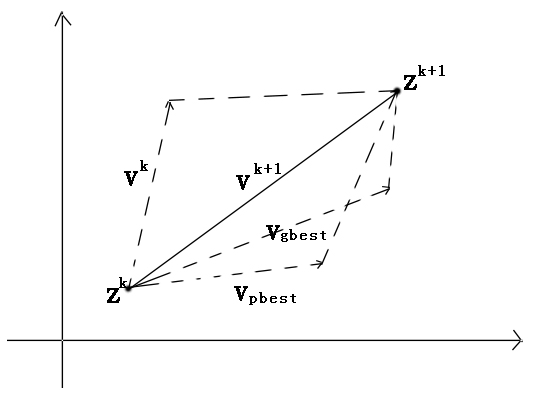算法流程：

1）开始
2）设定最大迭代次数（这里迭代次数人为设定，也可以自行根据条件来跳出循环）
3）设定群体个数（大群体搜索快计算慢，小群体搜索慢计算快）
4）初始化每个粒子的初始位子z
5）初始化每个粒子的初始速度v
6）计算每个粒子的初始位置的适应值fun（z），通过比较粒子间的适应值来选择初始群体最优位置gbest
7）将每个粒子各自的初始位置赋值给它们的pbest
8）k=0
9）k=k+1
10）根据速度更新公式更新每个粒子的新速度v
11）根据位置更新公式更新每个粒子的新位置z
12）每个粒子通过对比新旧位置更新pbest
13）更新粒子群体的最优位置gbest
14）从（9）开始迭代，直到达到迭代次数后跳出循环

算法效果展示：

输入函数f(x) = -1/2*((x1-50)(x1-50)+(x2-25)(x2-25)) , 我们很容易知道其最值为0，分别为x1=50和x2=25的时候：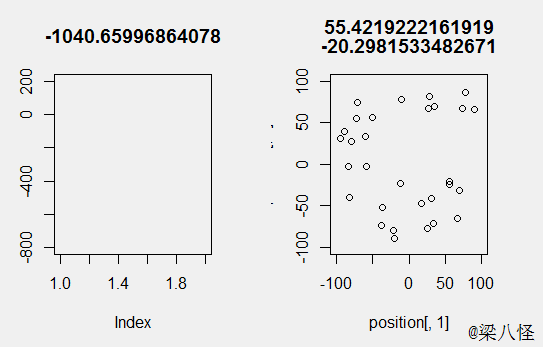输入函数f(x) = -1/2*(x1*x1+x2*x2+x3*x3) , 我们很容易知道其最值为0，分别为x1=0,x2=0,x3=0的时候：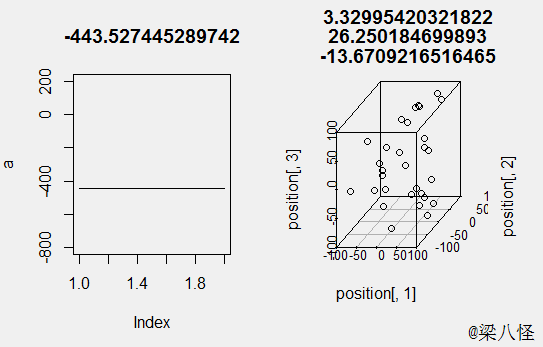R代码

PSO<-function(circle = 1000,biomass = 1000,c1,c2,position_range,vector_range,FUN,purpose = 'max'){
dimension<-nrow(position_range);#计算维度
position <- matrix(nrow = biomass,ncol = dimension)#初始化位置
for (i in 1:biomass) {
position[i,]<-apply(position_range, 1, FUN = function(x){
return( runif(n = 1,min = x,max= x))
})
}

vector <- matrix(nrow = biomass,ncol = dimension)##初始化速度
for (i in 1:biomass) {
vector[i,]<-apply(vector_range, 1, FUN = function(x){
return( runif(n = 1,min = x,max= x))
})
}

pbest<-position;#初始化每个粒子最优点

fun<-match.fun(FUN)

gbest<-matrix(data = rep(x = position[which(adopter == match.fun(purpose)(adopter,na.rm = T)),],biomass),nrow = biomass,byrow = T)

for (i in 1:circle) {
vector<-vector+c1*runif(n = 1,min = 0,max = 1)*(pbest-position)+c2*runif(n = 1,min = 0,max = 1)*(gbest-position)#更新速度
temp<-position+vector#重新计算位置

for (i in 1:dimension) {#控制粒子的位置和速度在域内
temp[which(temp[,i]<position_range[i,1]),]<-position_range[i,1]
temp[which(temp[,i]>position_range[i,2]),]<-position_range[i,2]
vector[which(vector[,i]<vector_range[i,1]),]<-vector_range[i,1]
vector[which(vector[,i]>vector_range[i,2]),]<-vector_range[i,2]
}

if (purpose == 'max')#更新每个粒子的最优点
c<-which(apply(temp, 1, FUN =fun)>apply(position, 1, FUN =fun))
else if (purpose == 'min')
c<-which(apply(temp, 1, FUN =fun)<apply(position, 1, FUN =fun))
pbest[c,]<-temp[c,]

position<-temp#更新位置

gbest<-matrix(data = rep(x = position[which(adopter == match.fun(purpose)(adopter,na.rm = T)),],biomass),nrow = biomass,byrow = T)
}

return(list(best = gbest[1,],value = fun(gbest[1,])))
}
展开全文• 针对惯性权重改进策略大多采用同代粒子使用相同权重,忽略了粒子本身特点以及不同维上的有效信息,提出一种基于不同粒子不同维的动态自适应惯性权重粒子群算法(AWPSO)。在该算法中利用矢量运算分析粒子进化公式,用一种...
• 粒子群算法

2021-01-22 17:25:28
粒子群算法（1.初步了解） ​ 1995年,受鸟类捕食行为的启发,Kennedy和Eberhart正式提出了粒子群优化算法的概念。研究中发现,在鸟类捕食过程中,个体并不知道如何找到食物以及自身离食物到底有多远,为了找到食物,最...

粒子群算法（1.初步了解）

​ 1995年,受鸟类捕食行为的启发,Kennedy和Eberhart正式提出了粒子群优化算法的概念。研究中发现,在鸟类捕食过程中,个体并不知道如何找到食物以及自身离食物到底有多远,为了找到食物,最有效的搜索方式是搜索离食物源最近的个体的周围区域,这种搜索方式为粒子群算法中信息共享机制提供了生物行为基础。同时,在认知过程中,每个个体都会建立自身的信念,同时观察其他个体的信念,当发现其他个体的信念更加优秀时,个体会进行相应的调整以提高自身的搜索能力,粒子群算法中的学习机制即是受这种行为的启发而产生的。

​ 简单例子说明大致思想：一只位于A处的小鸟在寻找食物的过程中它发现的最好的食物是位于B处的几粒小麦，而它的伙伴们们发现的最好的食物是位于C处的100只虫子，但这只小鸟既不想错过小麦，也不想错过虫子，应次它就选择网AB与AC矢量和的方向飞，而在这个方向飞的过程中它可能会找到比100只虫子更好的食物，它就会告诉其他伙伴，并调整自己的方向。

​ 一群群个体合作不仅能改进它们在某件任务上的集合性能而且还能提高每一个个体的性能,这正是粒子群优化(Particle swarm optimization)的基础.我们不仅在动物的行为中清楚看到粒子群优化的原理,在人类的行为中也能看到.当我们试图改进在某个任务上的性能时,会根据一些基本观点调整我们的方法.

• 惯性.我们往往会保留在过去已证明是成功的那些旧的方式。“我总是这样做,所以还会继续这样做”

• 受社会的影响.我们听到他人的成功后会试图仿照他人的方法.我们可能从书籍,或者互联网,或者报纸读到他人成功的事迹.“如果那样做对他们管用,对我可能也管用.”

• 受邻居的影响.我们从与自己亲近的人那里学到的最多、受朋友的影响会比受社会的影响更多.我们会与他人分享成功和失败的故事,并因为这些交往修正我们的行为.与互联网上亿万富翁的遥远的故事相比,百万富翁邻居或侄儿的投资建议对我们的影响更大.

​ 粒子群算法最初主要应用于连续函数的优化以及神经网络的训练,实践证明，多数情况下使用粒子群算法进行神经网络训练能够获得比BP算法更好的结果。已成功将粒子群算法应用于车间调度﹑旅行商(TSP)、整数规划等问题中。粒子群算法在同步发电机辨识、多目标优化、动态优化、聚类分析、游戏学习训练、生物信号检测与识别、新产品投人、目标检测以及广告优化等领域的应用均取得了一成果。

目前主要研究方向：理论研究、拓扑结构、参数选取与优化、其他算法的融合、算法的应用

1.算法框架

​ 使用粒子群算法求解实际优化问题时,一般首先要将待求解的问题空间映射为算法空间;然后进行初始化,通常的做法是随机生成一组均匀分布在搜索空间内的初始解;接着,根据搜索策略在算法参数的控制下在搜索区域内进行个体搜索，并产生一组待选解;以一定的接受准则(如确定性、概率性、混沌方式等)为依据对当前状态进行更新,如此反复迭代直到某种收敛标准得到满足;最后通过空间反变换,即将算法空间映射回问题空间,输出所求解问题的最优解。以上描述可以大致由下图来描述。2.算法设计步骤

（1）表示方案的确定

​ 也可以成为编码方案或粒子的表示方法，目前粒子群优化算法采用的编码方案大多为实数向量的形式,一般将解表示为粒子的位置向量。对于非数值优化问题而言,如生产调度问题等,如何通过合适的粒子表示方法来映射调度问题的解空间,是问题求解的关键环节。

（2）适应度值函数的确定

​ 适应度值函数用于评价待选解的质量,是唯一能够反映优化进程并引导其不断进行下去的参量。因此,对于特定的优化问题,根据问题的具体特征来选择合适的目标函数或代价函数来计算适应度值对于算法的求解质量有着重要的影响。

（3）控制参数的选取

​ 对于不同的算法模型,参数的选取直接关系到算法的求解性能。粒子群优化算法的主要控制参数包括:粒子种群规模、算法的最大迭代代数、惯性权重、学习因子以及其他一些辅助控制参数,如粒子的速度与位置的范围等。

（4）优化模型的选择

比较常见的模型包括带有惯性权重的粒子群算法模型、带有收缩因子的粒子群算法模型、采用拉伸技术的粒子群算法模型、二进制粒子群算法模型等。目前,在大多数优化问题中,带有线性递减惯性权重的粒子群算法模型得到了较多的应用,这主要归因于该模型可以有效地平衡全局寻优和局部寻优,从而提高优化效率。

（5）算法终止条件的确定

​ 与其他进化算法类似,粒子群优化算法通常采用最大迭代代数或凝滞代数作为算法的终止准则,即算法在迭代代数达到预先设定的最大值或者在搜索过程中全局最优适应度值持续一定的迭代代数不再发生明显改变时,迭代终止,算法寻优过程结束。

3.算法描述与分析

3.1算法基本模型

​ 在粒子群优化算法中,粒子的行为是一种共生合作的行为,每个粒子的搜索行为受到群体中其他粒子搜索行为的影响。同时,记忆了粒子过去的最好位置,具备对过去经验的简单学习能力。PSO先生成初始种群,即在可行解空间中随机初始化一群粒子,每个粒子都为优化问题的一个可行解,并由目标函数为之确定一个适应度值(fitness value)。每个粒子将在解空间中运动,并由速度决定其下一时刻的运动方向和距离。通常粒子将追随当前的最优粒子而动,并经逐代搜索最后得到最优解。在每一代中,粒子将跟踪两个极值,一个为粒子本身迄今找到的最优解 p b e s t p_{best} ,另一个为全种群迄今找到的最优解 p g b e s t p_{gbest}

​ 粒子的维度与解空间的维度一致，根据个体适应度来判断粒子的优劣，粒子根据自己的飞行经验(单个粒子自己找到的局部最优解 p b e s t pbest )和群体的飞行经验（全部粒子的全局最优解 g b e s t gbest ）来确定自身的飞行速度﹐调整自己的飞行轨迹,向最优点靠拢。粒子根据以下公式更新自己的速度和位置:

粒子i的第d维速度更新公式:
V i d k = w V i d k − 1 + c 1 r 1 ( p b e s t i d − x i d k − 1 ) + c 2 r 2 ( g b e s t d − x i d k − 1 ) V_{id}^k=wV_{id}^{k-1}+c_1r_1(pbest_{id}-x_{id}^{k-1})+c_2r_2(gbest_{d}-x_{id}^{k-1})
由以上公式可见,粒子的飞行轨迹由三部分组成:第一部分为粒子的运动惯性,包含粒子本身原有的速度 V i d k − 1 V_{id}^{k-1} 信息;其第二部分为“认知部分”,因为这部分考虑粒子自身的经验,通过与自己经历最佳位置 p b e s t pbest 的距离反映出来;第三部分是“社会部分”,表示粒子间的社会信息共享,通过与群体最好位置 g b e s t gbest 的距离来反映。 r 1 r_1 r 2 r_2 是在[0,1]的随机数; w , c 1 , c 2 w,c_1,c_2 是控制这三部分的权重，其中 w w 称为惯性权重, c 1 c_1 c 2 c_2 为加速因子，它们的含义如下：

​ (1)惯性权值 w w :控制粒子飞行速度变化。当w取值较大时,粒子飞行速度变化幅度较大,全局寻优能力强,局部寻优能力弱;反之当w取值较小时局部寻优能力强,全局寻优能力弱。选择一个合适的w可以平衡全局和局部搜索能力,这样可以以最少的迭代次数找到最优解。实验发现PSO的惯性权重在[0.9,1.2]具有较好的性能,还发现惯性权重从1.4减少到0要比用固定的好。这是因为开始较大的惯性权重可以遍历比较大的范围,后来小的权重有较好的局部搜索能力。

​ 线性递减权值：
w = w m a x − ( w m a x − w m i n ) ∗ r u n r u n m a x w=w_{max}-(w_{max}-w_{min})*\frac{run}{run_{max}}
r u n m a x 最 大 惯 性 权 重 , r u n m i n 最 小 惯 性 权 重 ， r u n 当 前 迭 代 次 数 ， r u n m a x 为 算 法 迭 代 总 次 数 run_{max}最大惯性权重,run_{min}最小惯性权重，run当前迭代次数，run_{max}为算法迭代总次数 ,可以使得粒子群算法在初期具有较强的全局收敛能力，而晚期其有较强的局部收敛能力。

​ (2)加速常数 c 1 c_1 ，和 c 2 c_2 :代表了粒子向自身极值和全局极值推进的随机加速权值，当 c 1 c_1 为0时，表示每个粒子都过于“奉献”，不相信自己的发现，只相信群体的发现，这样虽然有能力达到新的搜索空间,收敛速度比标准算法快,但碰到复杂问题比标准算法更容易陷入局部极值。当 c 2 c_2 为0时，这表示，每个粒子都只相信自己，一点也不相信群体，此时得到最优解的概率很小。如果 c 1 = c 2 = 0 c_1=c_2=0 说明粒子以当前速度飞行直到边界

粒子i的第d维位置更新公式：
x i d k = x i d k − 1 + V i d k x_{id}^k=x_{id}^{k-1}+V_{id}^k
其中 V i d k V_{id}^k 为第k次迭代粒子i飞行速度矢量的第d维分量； x i d k x_{id}^k 为第k次迭代粒子i位置矢量的第d维分量。

​ 为了降低搜索过程中粒子离开搜索空间的可能性,通常第d维的位置变化限定在 [ X m i n , X m a x ] [X_{min},X_{max}] 内;速度变化范围也限定在 [ − v m i n , v m a x ] [-v_{min},v_{max}] 内,其中最大速度 v m a x v_{max} 的选择不应超过的粒子宽度范围,如果 v m a x v_{max} 太大,可能飞过最优解的位置;如果太小，可能降低粒子的全局搜索能力。群体规模一般来说不用取的太大，一般几十个粒子就足够用了。如针对多目标优化等比较难的问题或者某些特定的问题,粒子数可以取到100～200个。粒子的维度由优化问题所决定,也就是解空间的维度。

3.2算法流程

​ (1)设定相关参数 c 1 , c 2 , w c_1 ,c_2 ,w 和粒子个数,并初始化种群,随机生成群体中的粒子候选解的位置和速度。
​ (2)逐一评价种群中的个体,即计算每个粒子对应的适应度值( fitness value),用以衡量此粒子的“优秀程度”。
​ (3)对于每个个体的适应度值,将其与自身所经历过的最好位置 p b e s t pbest 值进行比较,若较好,则将其作为当前的最好位置;将其适应度值与群体所经历的历史最优值 g b e s t gbest 的适应度值进行比较,若较好,则将其作为全局最好位置。
​ (4)按照上述速度及位置更新公式计算每个粒子新的时刻的速度和位置。
​ (5)检查结束条件是否满足。如果满足就结束计算,否则转至步骤(2)。

流程图如下：3.3全局模型和局部模型

​ 以上算法描述中,粒子跟踪两个极值,自身极值 p b e s t pbest 和种群全局极值 g b e s t gbest ,称为全局版本(global version)PSO。另一种为局部版本(local version)PSO,指粒子除了追随自身极值 p b e s t pbest 外,不跟踪全局极值 g b e s t gbest ,而是追随拓扑近邻粒子当中的局部极值 l b e s t lbest 。在该版本中,每个粒子需记录自己和它邻居的最优值,而不需要记录整个群体的最优值。例如,邻居大小为,则第i个粒子只须比较自己适应度值和第i—1和第i＋1粒子的适应度值大小。因此,局部版本的粒子群优化算法粒子的速度更新公式如下：
V i d k = w V i d k − 1 + c 1 r 1 ( p b e s t i d − x i d k − 1 ) + c 2 r 2 ( l b e s t d − x i d k − 1 ) V_{id}^k=wV_{id}^{k-1}+c_1r_1(pbest_{id}-x_{id}^{k-1})+c_2r_2(lbest_d-x_{id}^{k-1})
其中 l b e s t d lbest_d 为局部最优点

​ 比较全局和局部版本两种算法,可以注意到,它们收敛速度和跳出局部最优的能力有所差异。由于全局拓扑结构中所有粒子都信息共享,粒子向当前最优解收敛的趋势非常显著,因而全局模型通常收敛到最优点的速度较局部结构快，但更易陷入局部极小,表现为整个种群一致收敛到当前第一个较好的解。对此更好的理解,可以考虑模拟退火的成功之处,在其中较差的一些解有时也被保留。局部拓扑结构模型则允许粒子与其邻居比较当前搜索到的最优位置,从而相互之间施加影响,即便其值比种群最好值要差,该影响可以使较差个体进化为较好的个体。

3.4同步模式与异步模式

​ 粒子群优化算法在求解优化问题时,全局最优值的更新分为两种模式——同步模式与异步模式。下面对两种模式进行介绍。
​ 同步模型(synchronization)中,每代所有粒子都并行移动后,再选择种群最优粒子。而在异步(asynchronization)模型中,当粒子的任何一个邻居更新后,都与最优粒子进行比较,以便及时更新最优粒子。
​ 研究发现,在多数情况下,异步模型比同步模型能更快地找到问题的最优解。

展开全文算法 粒子群算法
• 二、粒子群算法的具体表述 上面罗嗦了半天，那些都是科研工作者写论文的语气，不过，PSO的历史就像上面说的那样。下面通俗的解释PSO算法。 PSO算法就是模拟一群鸟寻找食物的过程，每个鸟就是PSO中的粒子，也就是...
• 改进粒子群算法在暴雨强度公式参数优化中的应用.pdf
• 粒子群算法（PSO） C

千次阅读 2018-09-21 19:56:02
粒子群算法的核心的两个公式为： 速度更新公式： Vid(k+1)=wVid(k)+c1r1(Pid(k)-Xid(k))+c2r2(Pgd(k)-Xid(k))* 位置更新公式： Xid(k+1) = Xid(k) + Vid(k+1) w称之为惯性权重，体现的是粒子继承先前速度的能力。 ...

粒子群算法的核心的两个公式为：
速度更新公式：
Vid(k+1)=wVid(k)+c1r1*(Pid(k)-Xid(k))+c2r2(Pgd(k)-Xid(k))
位置更新公式：
Xid(k+1) = Xid(k) + Vid(k+1)

w称之为惯性权重，体现的是粒子继承先前速度的能力。 经验表明：一个较大的惯性权重有利于全局搜索，而一个较小的惯性权重则更有利于局部搜索。

为了更好地平衡算法的全局搜索能力与局部搜索能力，Shi.Y提出了线性递减惯性权重(LDIW)
即：w(k) = w_end + (w_start- w_end)*(Tmax-k)/Tmax。*其中w_start 为初始惯性权重，w_end 为迭代至最大次数时的惯性权重;k为当前迭代次数， Tmax为最大迭代次数。

一般来说，w_start=0.9,w_end=0.4时，算法的性能最好。这样随着迭代的进行，惯性权重从0.9递减到0.4,迭代初期较大的惯性权重使算法保持了较强的全局搜索能力。而迭代后期较小的惯性权重有利于算法进行更精确的局部搜索。线性惯性权重，只是一种经验做法，常用的惯性权重还包括 以下几种。*

(3) w(k) = w_start - (w_start-w_end)(k/Tmax)^2
(4) w(k) = w_start + (w_start-w_end)
(2k/Tmax - (k/Tmax)^2)
(5) w(k) = w_end
(w_start/w_end)^(1/(1+c*k/Tmax)) ,c为常数，比如取10等。

本例的目的就是比较这5种不同的w取值，对于PSO寻优的影响。比较的方法为每种w取值，重复实验若干次(比如100次),比较平均最优解的大小，陷入次优解的次数，以及接近最优解的次数。 这样对于5种方法的优劣可以有一个直观的比较。

代码：

/*
* 本例的寻优非线性函数为
* f(x, y) = sin(sqrt(x ^ 2 + y ^ 2)) / (sqrt(x ^ 2 + y ^ 2)) + exp((cos(2 * PI*x) + cos(2 * PI*y)) / 2) - 2.71289
* 该函数有很多局部极大值点，而极限位置为(0, 0), 在(0, 0)附近取得极大值
*/

#include<stdio.h>
#include<stdlib.h>
#include<math.h>
#include<time.h>
#define c1 1.49445 //加速度因子一般是根据大量实验所得
#define c2 1.49445
#define maxgen 300  // 迭代次数
#define repeat 100 // 重复实验次数
#define sizepop 20 // 种群规模
#define popmax 2 // 个体位置最大取值
#define popmin -2 // 个体位置最小取值
#define Vmax 0.5 // 速度最大值
#define Vmin -0.5 //速度最小值
#define dim 2 // 粒子的维数
#define w_start 0.9
#define w_end 0.4
#define PI 3.1415926 //圆周率

double pop[sizepop][dim]; // 定义种群数组
double V[sizepop][dim]; // 定义种群速度数组
double fitness[sizepop]; // 定义种群的适应度数组
double result[maxgen];  //定义存放每次迭代种群最优值的数组
double pbest[sizepop][dim];  // 个体极值的位置
double gbest[dim]; //群体极值的位置
double fitnesspbest[sizepop]; //个体极值适应度的值
double fitnessgbest; // 群体极值适应度值
double genbest[maxgen][dim]; //每一代最优值取值粒子

double func(double * arr);	//适应度函数
void pop_init(void);	 // 种群初始化
double * max(double * fit, int size);	// max()函数定义
void PSO_func(int n);	// 迭代寻优，传入的参数为一个整数，取值为1到5,分别代表5种不同的计算w的方法

int main(void)
{
clock_t start, finish; //程序开始和结束时间
start = clock(); //开始计时
srand((unsigned)time(NULL)); // 初始化随机数种子
for (int i = 1; i <= 5; i++)
{
int near_best = 0; // 接近最优解的次数
double best_sum = 0; // 重复最优值求和
double best = 0; // 重复实验得到的最优解
for (int j = 0; j < repeat; j++)    //repeat为迭代次数
{
PSO_func(i); // 第i种w参数取值，表示采用第i种惯性权重w的线性递减方式，完成粒子群搜索
double * best_fit_index = max(result, maxgen);  //result是存放每次迭代最优值的数组，maxgen迭代次数
double best_result = *(best_fit_index + 1); //最优解
if (best_result > 0.95)
near_best++;
if (best_result > best)
best = best_result;
best_sum += best_result;
}
double average_best = best_sum / repeat; //重复实验平均最优值
printf("w参数的第%d种方法:\n", i);
printf("重复实验%d次，每次实验迭代%d次,接近最优解的实验次数为%d次，求得最优值为:%lf,平均最优值为:%lf\n",
repeat, maxgen, near_best, best, average_best);
}
finish = clock(); //结束时间
double duration = (double)(finish - start) / CLOCKS_PER_SEC; // 程序运行时间
printf("程序运行耗时:%lf\n", duration);
system("pause");
return 0;
}

double func(double * arr)	//适应度函数
{
double x = *arr; //x 的值
double y = *(arr + 1); //y的值
double fitness = sin(sqrt(x*x + y*y)) / (sqrt(x*x + y*y)) + exp((cos(2 * PI*x) + cos(2 * PI*y)) / 2) - 2.71289;
return fitness;

}

void pop_init(void)	 // 种群初始化
{
for (int i = 0; i<sizepop; i++)
{
for (int j = 0; j<dim; j++)   //dim为粒子维数2
{
pop[i][j] = (((double)rand()) / RAND_MAX - 0.5) * 4; //-2到2之间的随机数
V[i][j] = ((double)rand()) / RAND_MAX - 0.5; //-0.5到0.5之间
}
fitness[i] = func(pop[i]); //计算适应度函数值
//pop[i]表示二维数组pop的pop[i]的地址
}
}

double * max(double * fit, int size)	// 求适应度函数值最大值及其位置
{
int index = 0; // 初始化序号
double max = *fit; //初始化最大值为数组第一个元素
static double best_fit_index;
for (int i = 1; i<size; i++)
{
if (*(fit + i) > max)
max = *(fit + i);
index = i;
}
best_fit_index = index;
best_fit_index = max;
return best_fit_index;
}

void PSO_func(int n)	// 迭代寻优，传入的参数为一个整数，取值为1到5,分别代表5种不同的计算w的方法
{
pop_init();
double * best_fit_index; // 用于存放群体极值和其位置(序号)
best_fit_index = max(fitness, sizepop); //求群体极值
int index = (int)(*best_fit_index);
// 群体极值位置
for (int i = 0; i<dim; i++)    //dim为粒子维数2
{
gbest[i] = pop[index][i];
}
// 个体极值位置
for (int i = 0; i<sizepop; i++)
{
for (int j = 0; j<dim; j++)
{
pbest[i][j] = pop[i][j];
}
}
// 个体极值适应度值
for (int i = 0; i<sizepop; i++)
{
fitnesspbest[i] = fitness[i];
}
//群体极值适应度值
double bestfitness = *(best_fit_index + 1);
fitnessgbest = bestfitness;

//迭代寻优
for (int i = 0; i<maxgen; i++)
{
for (int j = 0; j<sizepop; j++)
{
//速度更新及粒子更新
for (int k = 0; k<dim; k++)
{
// 速度更新
double rand1 = (double)rand() / RAND_MAX; //0到1之间的随机数
double rand2 = (double)rand() / RAND_MAX;
double w;
double Tmax = (double)maxgen;  //迭代次数
switch (n)
{
case 1:
w = 1;
case 2:
w = w_end + (w_start - w_end)*(Tmax - i) / Tmax;
case 3:
w = w_start - (w_start - w_end)*(i / Tmax)*(i / Tmax);
case 4:
w = w_start + (w_start - w_end)*(2 * i / Tmax - (i / Tmax)*(i / Tmax));
case 5:
w = w_end*(pow((w_start / w_end), (1 / (1 + 10 * i / Tmax))));
default:
w = 1;
}
V[j][k] = w*V[j][k] + c1*rand1*(pbest[j][k] - pop[j][k]) + c2*rand2*(gbest[k] - pop[j][k]);
//速度修正
if (V[j][k] > Vmax)
V[j][k] = Vmax;
if (V[j][k] < Vmin)
V[j][k] = Vmin;
// 粒子更新
pop[j][k] = pop[j][k] + V[j][k];
//位置修正
if (pop[j][k] > popmax)
pop[j][k] = popmax;
if (pop[j][k] < popmin)
pop[j][k] = popmin;
}
fitness[j] = func(pop[j]); //新粒子的适应度值
}
for (int j = 0; j<sizepop; j++)
{
// 个体极值更新
if (fitness[j] > fitnesspbest[j])
{
for (int k = 0; k<dim; k++)
{
pbest[j][k] = pop[j][k];
}
fitnesspbest[j] = fitness[j];
}
// 群体极值更新
if (fitness[j] > fitnessgbest)
{
for (int k = 0; k<dim; k++)
gbest[k] = pop[j][k];
fitnessgbest = fitness[j];
}
}
for (int k = 0; k<dim; k++)
{
genbest[i][k] = gbest[k]; // 每一代最优值取值粒子位置记录
}
result[i] = fitnessgbest; // 每代的最优值记录到数组
}
}

运行结果：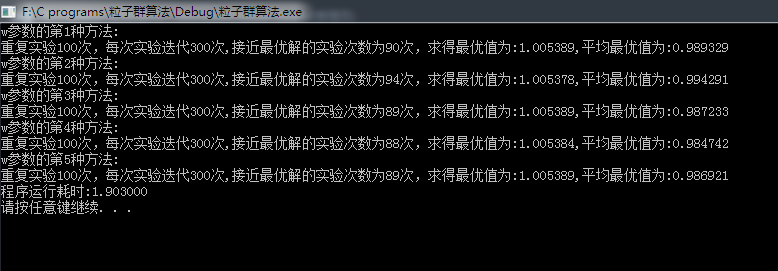展开全文C
• 基于粒子群算法的综合暴雨强度公式(英文).pdf
• 最优化算法之粒子群算法（PSO）

万次阅读 多人点赞 2018-08-03 10:26:45
一、粒子群算法的概念   粒子群优化算法(PSO：Particle swarm optimization) 是一种进化计算技术（evolutionary computation）。源于对鸟群捕食的行为研究。粒子群优化算法的基本思想：是通过群体中个体之间的...粒子群算法
• 粒子群算法(pso)标准测试函数验证程序。在一个m文件中包括了目前文献中用于验证的7个标准测试函数(Ackley等)、三维动态显示，粒子过分集中时打散等功能。旨在为学习和研究者pso算法的同仁提供一个功能较为完备、简单...
• 粒子群算法及其在暴雨强度公式参数优化中的应用.pdf
• 改进粒子群算法在暴雨强度公式参数优化中的应用 (1).pdf
• Matlab下的超详细的量子粒子群算法程序！有源码量子粒子群
• 粒子群算法反求割离井公式中的水文地质参数.pdf
• 优化算法——粒子群优化介绍 1. 基本概念 粒子群优化算法(PSO：Particle swarm optimization) 是一种进化计算技术（evolutionary computation）。源于对鸟群捕食的行为研究。粒子群优化算法的基本思想：是通过群体中...算法
• 8.粒子群算法（理论部分）

千次阅读 2020-09-22 22:27:02
本文主要介绍基础粒子群算法的主要理论，并简单介绍自适应权重分配与压缩因子的用法（即速度更新公式的三个系数改进）。 实际上粒子群算法经历了数十年发展，衍生出的改进算法多种多样，这里就不再过多介绍。下面...算法 粒子群算法 数学建模
• 粒子群算法【摘要】：粒子群算法(pso)是一种新兴的基于群体智能的启发式全局搜索算法，具有易理解、易实现、全局搜索能力强等特点，倍受科学与工程领域的广泛关注，已得到广泛研究和应用。为了进一步推广应用粒子群...
• 粒子群优化算法

千次阅读 多人点赞 2018-12-02 18:23:02
文章目录粒子群优化算法1、简介2、思想粒子群优化算法分析粒子群优化算法应用总结 粒子群优化算法 1、简介 粒子群算法，也称粒子群优化算法或鸟群觅食算法，英文为：Particle Swarm Optimization，缩写为 PSO， 是由...粒子群优化算法
• 针对约束边界粒子在边界区域搜索能力不足的问题, 提出一种基于自适应进化学习的约束多目标粒子群优化算法. 该算法根据不符合约束条件粒子的约束违反程度, 修正优化算法的进化学习公式, 提高算法在约束边界区域的搜索...
• matlab代码粒子群算法粒子群优化：最新技术 [pso]（使用python） PSO算法的基本变体是通过拥有一组候选解决方案（称为粒子）（称为“群”）来工作的。 群：这些粒子根据一些简单的公式在搜索空间中移动。 粒子的运动...
• 提出了一种基于量子粒子群的改进模糊聚类图像分割算法。针对FCM图像分割算法对聚类中心初始值比较敏感的缺点，利用量子粒子群优化算法强大的全局搜索能力寻找最优解，能够有效降低图像分割算法对初始值的依赖程度；...量子粒子群
• 粒子群算法的部分理论推导及推论证明，有数学基础的或者想自己推导公式及证明的可以下载学习，尤其是该算法的稳定性条件及收敛性分析。启发式算法...